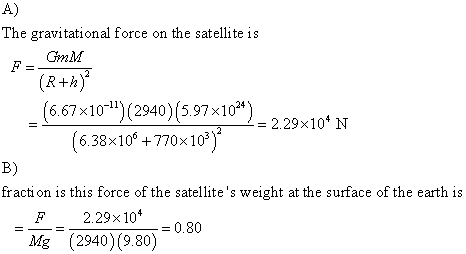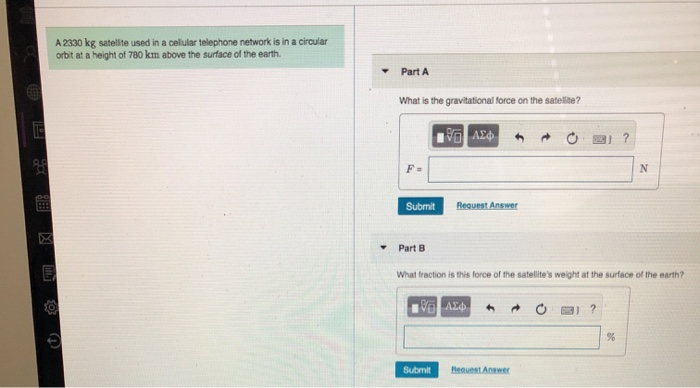Question

# A 2940 kg satellite used in a cellular telephone network is in a circular orbit at...

A 2940 kg satellite used in a cellular telephone network is in a circular orbit at a height of 770 kmabove the surface of the earth.

Part A

What is the gravitational force on the satellite?

Part B

What fraction is this force of the satellite's weight at the surface of the earth?#### Earn Coins

Coins can be redeemed for fabulous gifts.

Similar Homework Help Questions
• ### A 2250 kg satellite used in a cellular telephone network is in a circular orbit at...

A 2250 kg satellite used in a cellular telephone network is in a circular orbit at a height of 740 kmabove the surface of the earth. A) What is the gravitational force on the satellite? B) What fraction is this force of the satellite's weight at the surface of the earth?

• ### A 2560 kg satellite used in a cellular telephone network is in a circular orbit at...

A 2560 kg satellite used in a cellular telephone network is in a circular orbit at a height of 720 kmabove the surface of the earth. What is the gravitational force on the satellite? What fraction is this force of the satellite's weight at the surface of the earth?

• ### A 2710 kg satellite used in a cellular telephone network is in a circular orbit at...

A 2710 kg satellite used in a cellular telephone network is in a circular orbit at a height of 790 km above the surface of the earth. part a: What is the gravitational force on the satellite? part b: What fraction is this force of the satellite's weight at the surface of the earth? (convert to %)

• ### A 2830 kg satellite used in a cellular telephone network is in a circular orbit at...

A 2830 kg satellite used in a cellular telephone network is in a circular orbit at a height of 740 km above the surface of the earth. What is the gravitational force on the satellite? What fraction is this force of the satellite's weight at the surface of the earth?

• ### A 2660 kg satellite used in a cellular telephone network is in a circular orbit at...

A 2660 kg satellite used in a cellular telephone network is in a circular orbit at a height of 720 km above the surface of the earth. What is the gravitational force on the satellite?(Answer in N). What fraction is this force of the satellite's weight at the surface on the Earth?(Answer in %).

• ### A 2160 kg satellite used in a cellular telephone network is in a circular orbit at...

A 2160 kg satellite used in a cellular telephone network is in a circular orbit at a height of 800 km above the surface of the earth. What is the gravitational force on the satellite? What fraction is this force of the satellite's weight at the surface of the earth? I've looked at other problems but I'm not getting the correct answer, I've gotten 21168 for the first one and 0.998 for the second part.

• ### A 2330 kg satellite used in a celular telephone network is in a circular orbit at...A 2330 kg satellite used in a celular telephone network is in a circular orbit at a height of 780 km above the surface of the earth. Part A What is the gravitational force on the satellite? 90 AED + O2) Submit Request Answer Part B What fraction is this force of the satellite's weight at the surface of the earth? 90 AED + O2? Submit B est Answer

• ### A satellite used in a cellular telephone network has a mass of 2050 kg and is...

A satellite used in a cellular telephone network has a mass of 2050 kg and is in a circular orbit at a height of 880 km above the surface of the earth. Part A What is the gravitational force Fgrav on the satellite? Take the gravitational constant to be G = 6.67×10−11 N⋅m2/kg2 , the mass of the earth to be me = 5.97×1024 kg , and the radius of the Earth to be re = 6.38×106 m . part...

• ### A satellite used in a cellular telephone network has a mass of 2380 kg and is...

A satellite used in a cellular telephone network has a mass of 2380 kg and is in a circular orbit at a height of 850 km above the surface of the earth. Part A What is the gravitational force Fgrav on the satellite? Take the gravitational constant to be G = 6.67×10−11 N⋅m2/kg2 , the mass of the earth to be me = 5.97×1024 kg , and the radius of the Earth to be re = 6.38×106 m

• ### A 534-kg satellite is in a circular orbit about Earth at a height above Earth equal...

A 534-kg satellite is in a circular orbit about Earth at a height above Earth equal to Earth's mean radius. (a) Find the satellite's orbital speed. m/s (b) Find the period of its revolution. h (c) Find the gravitational force acting on it. N